+关注继续查看

# 再议 C 语言中的指针与数组（4）

## 二 数组与指针

### 2.1 指向数组的指针

# include<stdio.h>

int main(int argc,char *argv[]){
int a = {1,2,3,4,6,5,7,8,9,0};
int *p;
// p = a;
p = &a;
for (int i = 0; i < 10; i++){
printf("%d\t",*p);
p++;
}
return 0;
}
// 1       2       3       4       6       5       7       8       9       0

### 2.2 指向复合常量的指针

# include<stdio.h>

int main(int argc,char *argv[]){
int *p = (int []){1,2,3,4,6,5,7,8,9,0};
for (int i = 0; i < 10; i++){
printf("%d\t",*p);
p++;
}
return 0;
}
//1       2       3       4       6       5       7       8       9       0

# include<stdio.h>

int main(int argc,char *argv[]){
int *p = (int ){1,2,3,4,6,5,7,8,9,0};
for (int i = 0; i < 10; i++){
printf("%d\t",*p);
p++;
}
return 0;
}

### 2.3 通过指针对数组进行操作

# include<stdio.h>
# define N 10

int main(int argc,char *argv[]){
int a[N];
int *p;
for (p = a; p < a + N; p++){
scanf("%d",p);
}
for (p = a; p < a + N; p++){
printf("a[%d] = %d;\t ", p - a, *p);
}
printf("\n");
return 0;
}
/*
a = 1;        a = 2;       a = 3;       a = 4;       a = 5;       a = 66;      a = 7;       a = 7;       a = 8;       a = 9;
*/

### 2.4 指针与数组在函数定义中的使用

# include<stdio.h>

int main(int argc,char *argv[]){
unsigned int array[] = {3,5,6,7,1,2,0}, len;
int find_max(unsigned int [], int n);
len = sizeof array / sizeof array;
printf("%d\n",find_max(array,len));
return 0;
}

int find_max(unsigned int a[], int n){
int max = 0;
for (int i = 0; i < n; i++){
if ( a[i] > max)
max = a[i];
}
return max;
}
// 7

# include<stdio.h>

int main(int argc,char *argv[]){
unsigned int array[] = {3,5,6,7,1,2,0}, len;
int find_max(unsigned int [], int n);
// int find_max(unsigned int *, int n);
len = sizeof array / sizeof array;
printf("%d\n",find_max(array,len));
return 0;
}

int find_max(unsigned int *a, int n){
int max = 0;
for (int i = 0; i < n; i++){
if ( a[i] > max)
max = a[i];
}
return max;
}
// 7

### 2.5 多维数组与指针

# include<stdio.h>

int main(int argc,char *argv[]){
int *p, array = {{12,43,11},{10,56,89}};
for (p = &array; p <= &array; p++)
printf("array[%d][%d]: %d\n",(p - &array)/3, (p - &array)%3, *p);
return 0;
}
/*
array: 12
array: 43
array: 11
array: 10
array: 56
array: 89
*/

# include<stdio.h>

int main(int argc,char *argv[]){
int *p, array = {{12,43,11},{10,56,89}};
for (p = array; p <= &array; p++)
printf("array[%d][%d]: %d\n",(p - &array)/3, (p - &array)%3, *p);
return 0;
}

# include<stdio.h>
# define ROWS 2
# define COLS 3

int main(int argc,char *argv[]){
int *p, array[ROWS][COLS] = {{12,43,11},{10,56,89}};
for (p = *array; p < *array + (ROWS * COLS); p++)
printf("array[%d][%d]: %d\n",(p - *array) / COLS, (p - *array) % COLS, *p);
return 0;
}
/*
array: 12
array: 43
array: 11
array: 10
array: 56
array: 89
*/

## 三 总结

3.1 在 C 语言中，指针与数组关系密切，既有联系又有区别。在实际的使用过程中要特别谨慎。

3.2 指针是 C 语言中的精华。作为一名 C 语言学习者，指针虽然比较坑，但是这个是无法逃避的，必须花大量的时间与精力去学习与理解。

3.3 指针常常会与数组发生关联，对指针的理解必须透彻。

3.4 鉴于笔者水平，文中可能存在若干谬误，希望大家能帮忙指出，谢谢！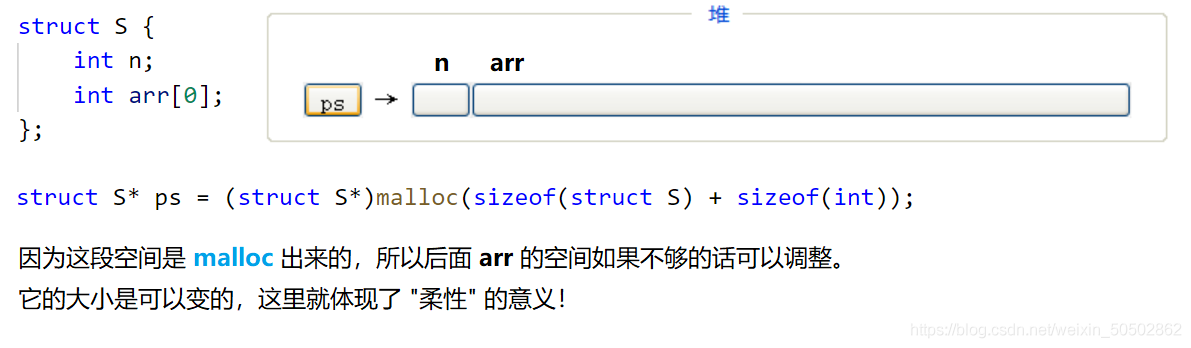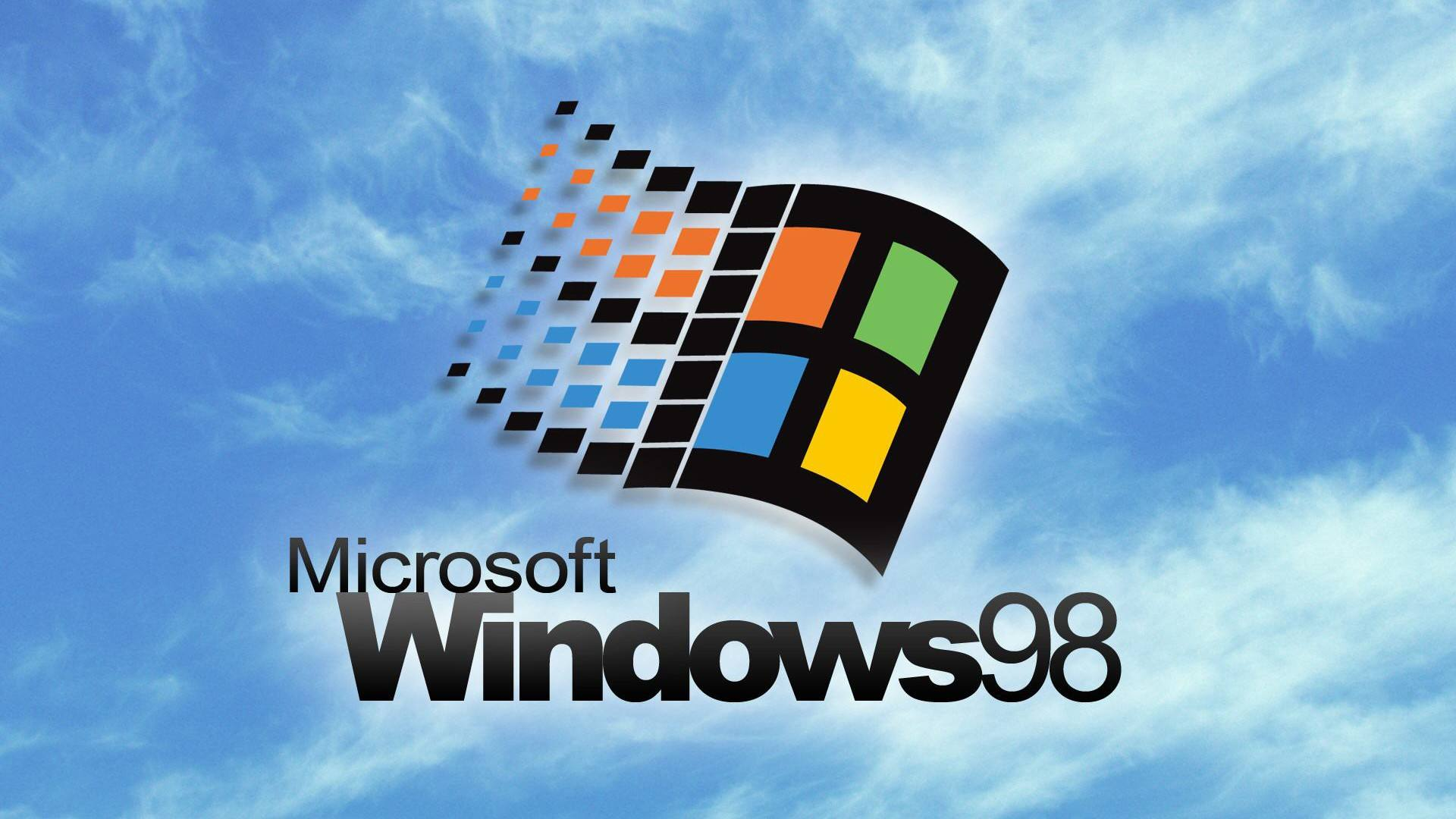【维生素C语言】第十五章 - 柔性数组（可变长数组）

0 0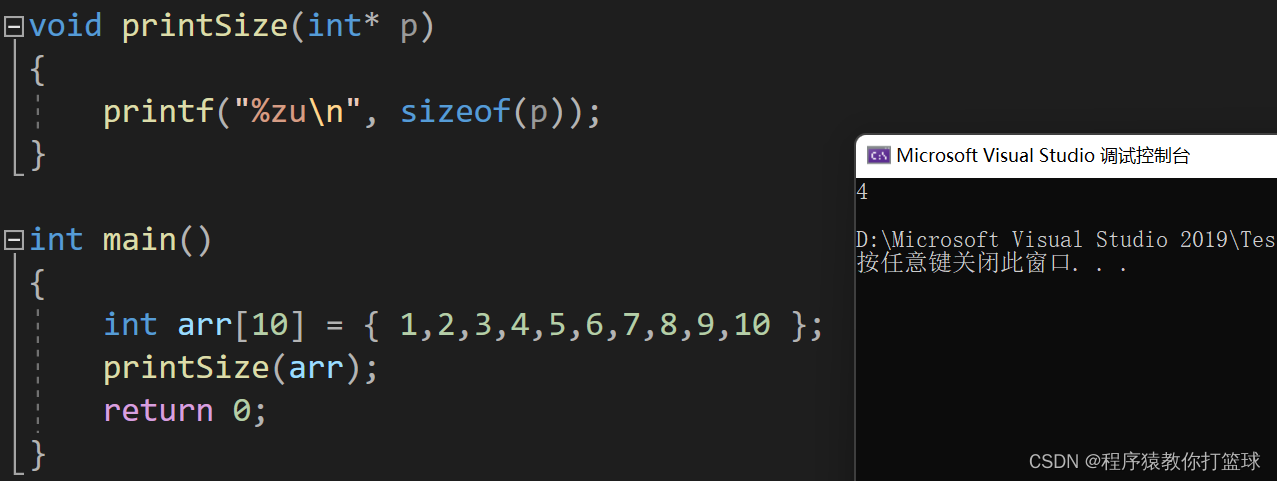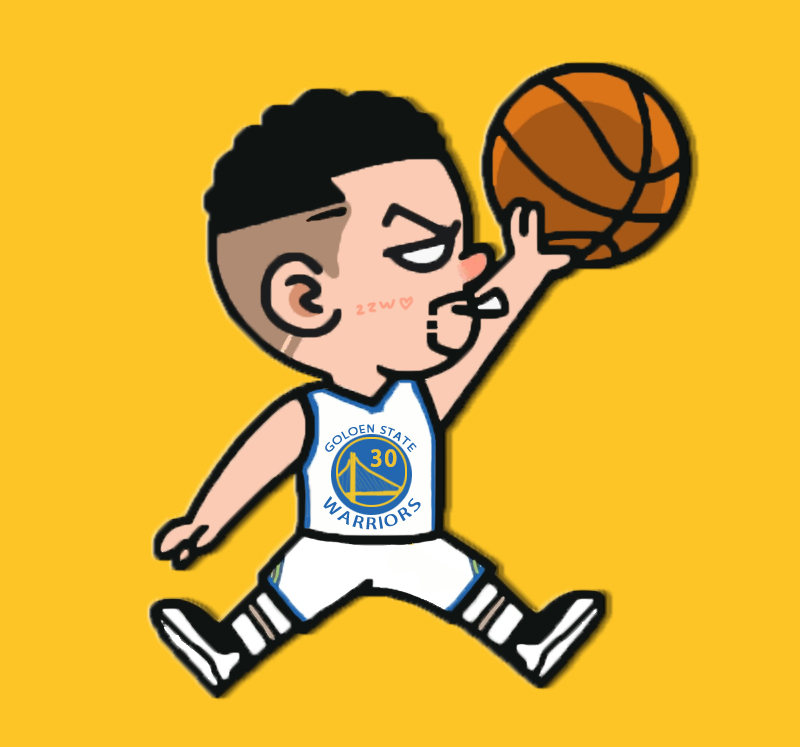【C语言】指针和数组的深入理解（第四期）（上）

0 0【C语言】指针和数组的深入理解（第四期）（下）

0 0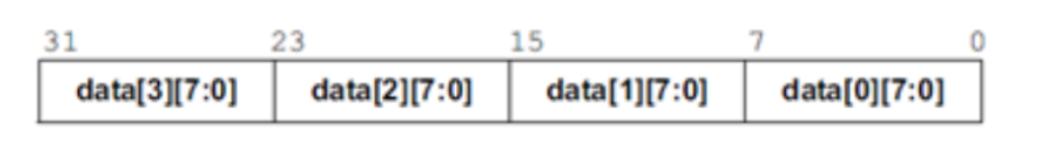SystemVerilog学习-05-数组
SystemVerilog学习-05-数组
0 0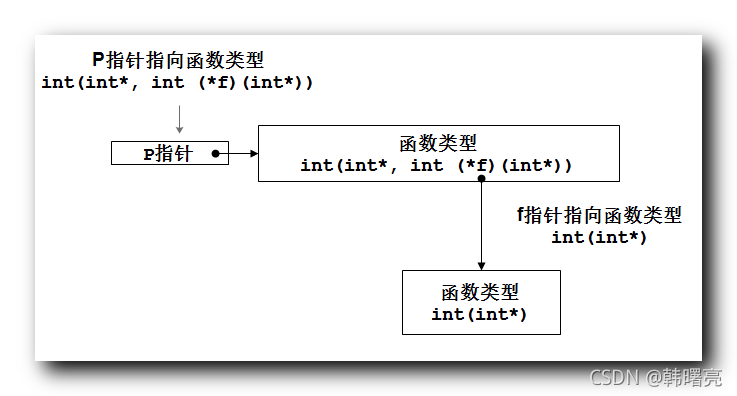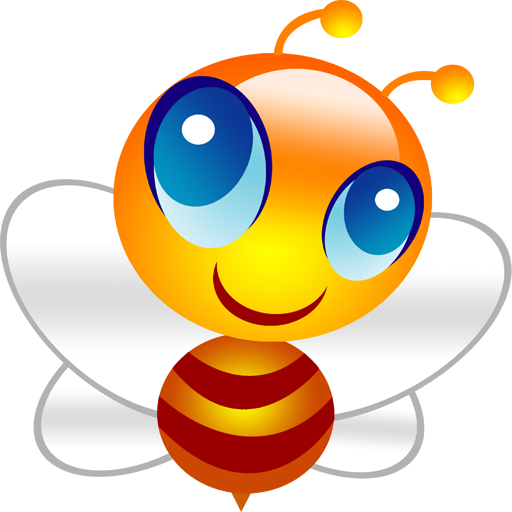【C 语言】指针数据类型 ( 指针步长 | 复杂指针阅读技巧 )
【C 语言】指针数据类型 ( 指针步长 | 复杂指针阅读技巧 )
0 0C语言核心之数组和指针详解
630 0《C和指针》一1.8　编程练习
1262 0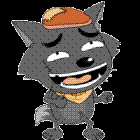707 0651 0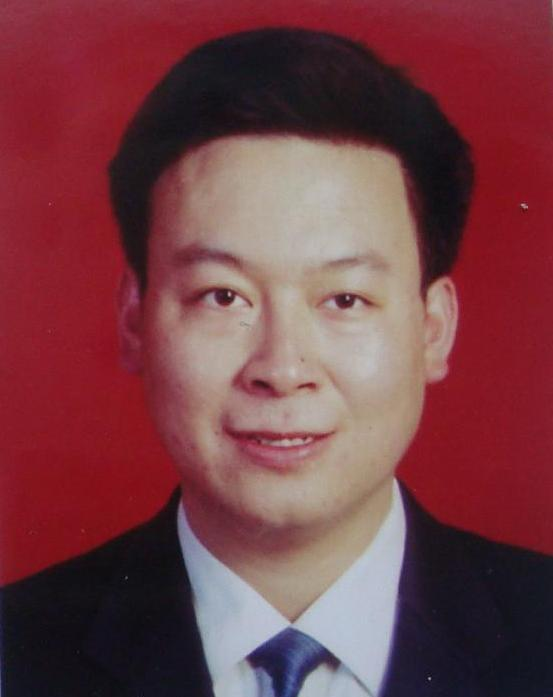363 0

Go语言核心编程 - 数据结构和算法158147

Go语言核心编程 - 基础语法、数组、切片、Map2721182